Function Repository Resource:

# EquivalentOrderings

Generalize Ordering by giving alternative orderings if there are equal items

 ResourceFunction["EquivalentOrderings"][list] return a list of all possible orderings of list considering the permutations of equal items. ResourceFunction["EquivalentOrderings"][list,n] return a list of all possible orderings of list considering the permutations of equal items, and limit each ordering to n items.

## Details and Options

All elements in list must accept the default comparison operator

## Examples

### Basic Examples (2)

Find the two ways of ordering this list:

 In:=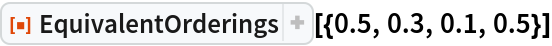Out=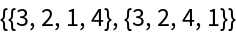If the orderings are limited to the first two elements, then there is only one way of ordering the list:

 In:=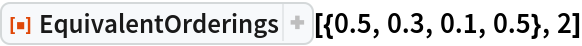Out=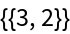EquivalentOrderings will work on the same non-numeric values as Ordering:

 In:=Out=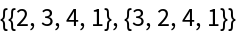Compare with Ordering:

 In:=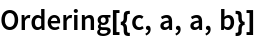Out=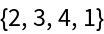### Scope (1)

Finding all possible orderings of a list of probabilities is helpful for considering alternative Huffman codes:

 In:=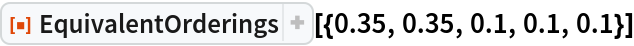Out=